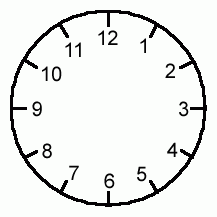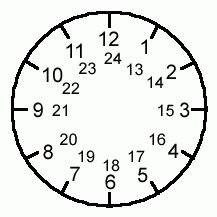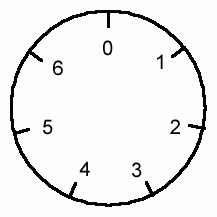# An Introduction to Modular Arithmetic

## Artikkel

Article by Vicky Neale

The best way to introduce modular arithmetic is to think of the face of a clock.The numbers go from $$1$$ to $$12$$, but when you get to "$$13$$ o'clock", it actually becomes $$1$$ o'clock again (think of how the $$24$$ hour clock numbering works). So $$13$$ becomes $$1$$, $$14$$ becomes $$2$$, and so on.

This can keep going, so when you get to "$$25$$ o'clock'', you are actually back round to where $$1$$ o'clock is on the clock face (and also where $$13$$ o'clock was too).So in this clock world, you only care where you are in relation to the numbers $$1$$ to $$12$$. In this world, $$1,13,25,37,…$$ are all thought of as the same thing, as are $$2,14,26,38,…$$ and so on.

What we are saying is "$$13=1+$$ some multiple of $$12$$", and "$$38=2+$$ some multiple of $$12$$", or, alternatively, "the remainder when you divide $$13$$ by $$12$$ is $$1$$" and "the remainder when you divide $$38$$ by $$12$$ is $$2$$''. The way we write this mathematically is $$13≡1 \bmod 12, 38≡2 \bmod 12$$, and so on. This is read as "$$13$$ is congruent to $$1 \bmod$$ (or modulo) $$12$$" and "$$38$$ is congruent to $$2 \bmod 12$$".

But you don't have to work only in $$\bmod 12$$ (that's the technical term for it). For example, you could work $$\bmod 7$$, or $$\bmod 46$$ instead if you wanted to (just think of clocks numbered from $$1$$ to $$7$$ and $$1$$ to $$46$$ respectively; every time you get past the biggest number, you reset to $$1$$ again).Let's go back to the normal clock face with the numbers $$1$$ to $$12$$ on it for a moment. Mathematicians usually prefer to put a $$0$$ where the $$12$$ would normally be, so that you would usually write (for example) $$24≡0$$ $$\bmod 12$$ rather than $$24≡12 \bmod 12$$, although both of these are correct. That is, we think of a normal clock face as being numbered from $$0$$ to $$11$$ instead. This makes sense: we'd normally say that $$24$$ leaves a remainder of $$0$$ when we divide by $$12$$, rather than saying it leaves a remainder of $$12$$ when we divide by $$12$$!

Let's be a bit more formal. In general, if you are working in $$\bmod n$$ (where n is any whole number), we write $$a≡b \bmod n$$ if a and b leave the same remainder when you divide them by n. This is the same as saying that we write $$a≡b \bmod n$$ if n divides $$a−b$$. (Look at what we did earlier to see that this definition fits with our examples above.)

So far, we've only talked about notation. Now let's do some maths, and see how congruences (what we've described above) can make things a bit clearer.

Here are some useful properties. We can add congruences. That is, if $$a≡b \bmod n$$ and $$c≡d \bmod n$$, then $$a+c≡(b+d) \bmod n$$. Why is this? Well, $$a≡b \bmod n$$ means that $$a=b+kn$$, where k is an integer. Similarly, $$c≡d \bmod n$$ means that $$c=d+ln$$, where $$l$$ is an integer. So $$a+c=(b+kn)+(d+ln)=(b+d)+(k+l)n$$, so $$a+c≡(b+d) \bmod n$$. For example, $$17≡4 \bmod 13$$, and $$42≡3 \bmod 13$$, so $$17+42≡4+3≡7 \bmod 13$$. Note that both of the congruences that we're adding are mod n, and so is the answer - we don't add the moduli.

Now you prove that if $$a≡b$$$$\bmod n$$ and $$c≡d \bmod n$$ then $$a−c≡(b−d) \bmod n$$. Also, prove that we can do something similar for multiplication: if $$a≡b \bmod n$$ and $$c≡d \bmod n$$, then $$ac≡bd \bmod n$$. You can prove this in the same way that we used above for addition. Again, both of the congruences that we're multiplying are $$\bmod n$$, and so is the answer - we don't multiply the moduli. Can you come up with an example to disprove the claim that $$a≡b \bmod n$$ and $$c≡d \bmod m$$ means that $$ac≡bd \bmod mn$$?

Division is a bit more tricky: you have to be really careful. Here's an example of why. $$10≡2 \bmod 8$$. But if we "divide both sides by $$2$$", we'd have $$5≡1 \bmod 8$$, which is clearly nonsense! To get a true congruence, we'd have to divide the $$8$$ by $$2$$ as well: $$5≡1 \bmod 4$$ is fine. Why? Well, $$a≡b \bmod n$$ means that $$a=b+kn$$ for some integer $$n$$. But now this is a normal equation, and if we're going to divide a by something, then we have to divide all of the right-hand side by $$2$$ as well, including $$kn$$. In general, it's best not to divide congruences; instead, think about what they really mean (rather than using the shorthand) and work from there.

Things are quite special if we work $$\bmod p$$, where $$p$$ is prime, because then each number that isn't $$0 mod p$$ has what we call an inverse (or a multiplicative inverse , if we're being fancy). What that means is that for each $$a≢0 \bmod p$$, there is a $$b$$ such that $$ab≡1 \bmod p$$.

Let's think about an example. We'll work $$\bmod 7$$. Then really the only non-zero things are $$1,2,3,4,5$$ and $$6$$(because every other whole number is equivalent to one of them or $$0$$). So let's find inverses for them. Well, $$1$$ is pretty easy: $$1×1≡1 \bmod 7$$. What about $$2$$? $$2×4≡1 \bmod 7$$. So $$4$$ is the inverse of $$2$$. In fact, we can also see from this that $$2$$ is the inverse of $$4$$ - so that's saved us some work! $$3×5≡1 \bmod 7$$, so $$3$$ and $$5$$ are inverses. And finally, $$6×6≡1 \bmod 7$$, so $$6$$ is the inverse of itself. So yes, each of the non-zero elements $$\bmod 7$$ has an inverse. Try some primes out yourself: $$11$$ and $$13$$ are fairly small! If you're feeling confident, see whether you can discover which numbers have inverses $$\bmod 4$$, or $$\bmod 6$$, or $$\bmod 8$$. What about $$\bmod 15$$? Do you notice any patterns?

To prove this, things are going to get a tiny bit more tricky, so I'm going to save the proof for the end and first give an example of using congruences to do useful mathematics.

Suppose we're given the number $$11111111$$ and someone asks us whether it's divisible by $$3$$. We could try to actually divide it. But you probably know a much easier method: we add up the digits and see whether that's divisible by $$3$$. There's a whole article about this sort of divisibility test here. Let's prove this using congruence notation.

Suppose that our number is $$a_n10^n+a_{n−1}10^{n−1}+…+10_{a1+a0}$$, so it looks like $$a_na_{n−1}…a_1a_0$$. Then the sum of its digits is $$a_n+a_{n−1}+…+a_1+a_0$$. We'd like to prove that $$a_n10^n+a_{n−1}10^{n−1}+…+10_{a1+a0}$$ is divisible by $$3$$ if and only if $$a_n+a_{n−1}+…+a_1+a_0$$ divisible by $$3$$. Now we notice that $$10≡1 \bmod 3$$, so $$10×10≡1 \bmod 3$$, and more generally $$10^k≡1 \bmod 3$$ for all $$k$$. Using our results from earlier about adding and multiplying congruences, we discover

$$a_n10^n+a_{n−1}10^{n−1}+…+10a_1+a_0≡a_n+a_{n−1}+…+a_1+a_0 \bmod 3$$

So if our number is divisible by $$3$$ (that is, if $$a_n10^n+a_{n−1}10^{n−1}+…+10a_1+a_0≡0\bmod 3$$), then certainly so is the sum of its digits, and vice versa, as we wanted! The congruence notation hasn't really done any of the maths for us, but it's hopefully made it a bit easier to write out the proof clearly. See whether you can use the notation to prove any of the other divisibility tests in that article.

Now for the proof I promised you earlier. We're going to show that if $$a$$ and $$n$$ have no common factors, then $$a$$ has a multiplicative inverse $$\bmod n$$ (reminder: that means a number $$b$$ such that $$a×b≡1 \bmod n$$). In particular, if $$n$$ is prime, then its only factor apart from $$1$$ is itself, so saying "$$a$$ and $$n$$ share no common factors'' is just the same as saying "$$a$$ isn't divisible by $$n$$'', that is, $$a≢0 \bmod p$$: this is what we had above. I'm going to assume that you know about using Euclid's algorithm to solve equations of the form $$ax+by=1$$ where $$a$$ and $$b$$ have no common factors. There's a really good article explaining this here, so have a read, and then come back.

If you're reading this far, then hopefully you'll agree that if $$a$$ and $$n$$ share no common factors, then we can find $$x$$ and $$y$$ such that ax+ny=1. (The fancy name for this is Bezout's Theorem .) So we've got our x and y such that $$ax+ny=1$$. We can rewrite this as $$ax=1−yn$$, and now let's use the congruence notation from earlier: we have $$ax≡1 \bmod n$$. So now $$x$$ is the multiplicative inverse of $$a \bmod n$$, and we're done!

Here's a challenge: use this technique based on Euclid's algorithm to find the inverse of $$14 \bmod 37$$.

Understanding this is the beginning of a branch of mathematics called Number Theory, which contains some beautiful, fascinating and amazing theorems. Enjoy!

Ressursen er utviklet av NRICH

10